Next: Results and Discussion Up: Analysis Previous: Calculation of the Core Radius

# Fit Quality

Figure 5.5 displays a typical fitted muon precession signal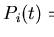in a rotating reference frame.

The fitted function passes through most of the data points. The insets to this figure contain residual plots which exhibit a clear oscillation, indicating that the fitted function describes the data imperfectly. Such plots offer a useful qualitative assessment of the accuracy of a fit.

Chi-squared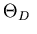provides a quantitative evaluation of the fit quality. It is defined as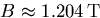(7.8)

where f(x) is the function fitted to the n measurements. A decrease inof at least one reveals a significantly improved fit, while a reduced chi-squaredmeans the fit is perfect. For the results reported in this thesis, the minimised reduced chi-squareds range betweenand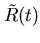. These fits are comparable in quality to those obtained for other superconductors through analysis of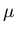SR data in the time domain. Use of a more accurate cutoff factor  than the simple Gaussianin expression (5.2) might supply better fits for the LuNi2B2C data discussed in this thesis. Figure 5.6 shows the dependence of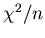on the penetration depth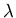in LuNi2B2C at several temperatures T, and Table 5.1 lists the number of degrees of freedom n for each of these temperatures. (The number of degrees of freedom n for fits at other temperatures is similar.)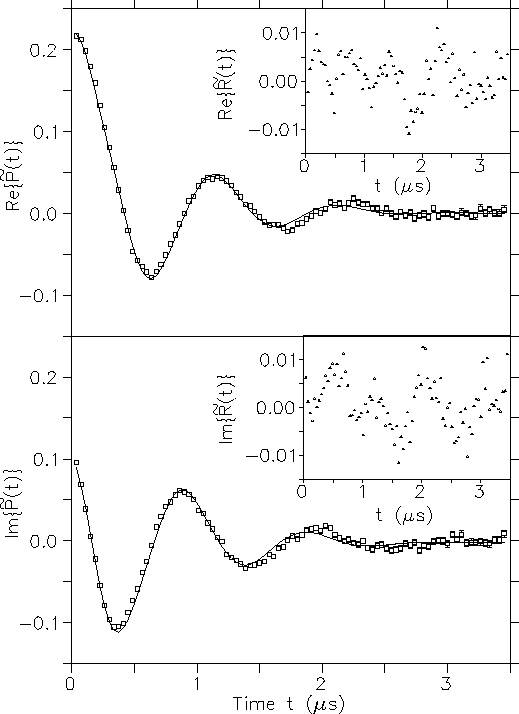Temperature T ( K) Number of data points n 2.6 556 5.5 550 8.5 547

For each set value of the penetration depththe parameters vary, in the way described in Section 5.3, untilis minimised. The error bars for fitted parameters reported in this thesis are the amount by which the parameter must change to raiseby one while the other parameters vary normally. At each temperature there is a clear minimum inas a function of penetration depth, which moves to higher values of the penetration depthat warmer temperatures. The shallowness of the minima is at least partly a consequence of compensation by the other free parameters, especially the nonlocality parameter C. Parameter C drops monotonically by two to three orders of magnitude over the displayed interval of increasing penetration depth. This substantial playoff comes from the factor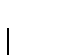appearing in the internal spatial field profile (5.2). The widening minima at higher temperatures T reflects the diminishing asymmetry of the internal field distribution n(B) as it approaches a Gaussian form. Figure 5.7 reveals howvaries with the nonlocality parameter C.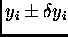At each temperaturegrows rapidly as Cdecreases below the location of the minimum. This clearly demonstrates the substantial improvement in fit quality achieved by incorporating nonlocal corrections into the London model. The slow growth inas the nonlocality parameter C increases away from the minimum further evidences the playoff between C and the penetration depth. The penetration depthfalls monotonically as the nonlocality parameter C rises over the plotted interval. The shallower minima at warmer temperatures Tagain stem from the more Gaussian-like internal field distribution n(B). Figure 5.8 displaysfor a range of vortex core radii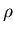at the same temperatures T.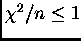Since the core radiusis a calculated rather than fitted parameter, this graph is constructed by minimisingat fixed values of the coherence length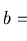and noting the corresponding core radii. The strongly linear relationship between the coherence lengthand the core radiusmakes this process possible. Similarly, altering the coherence lengthby its uncertainty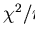and observing the consequent change in the core radiusproduces error bars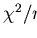for the core radius. This means of computation of the uncertaintyin the core radiusis far simpler and more tractable than one based on combining the uncertainties of the fitted parameters according to equations (5.2) and (5.10). At each temperature T thecurve has a single minimum, which moves to larger core radiias the temperature T rises. This behaviour of the core radius, and also the penetration depth, with temperature T is explored in more detail in the next chapter.Next: Results and Discussion Up: Analysis Previous: Calculation of the Core Radius
Jess H. Brewer
2001-10-31